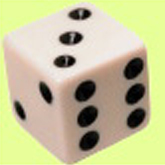# What Are Cubes And Cube Roots?The mathematical term 'cube' comes from the three-dimensional shape of the same name. A cube shape has three dimensions of length, width, and height, all equal and at angles of 90 to each other. Put another way, a cube is as high as it is wide as it is long. The mathematical cube of a number comes from the shape of a cube by the number of standard-sized cubes that it contains. For example, a cube that measures 9 centimeters on a side contains 729 smaller cubes that are each 1 centimeter on a side. This can be demonstrated by building the cube using sugar cubes. Start by making a layer of sugar cubes that is 9 sugar cubes long and 9 sugar cubes wide. Then build 8 more layers just like it, one on top of the other, until there are a total of 9 layers. Now count the number of single sugar cubes you used to build the large 9-layer cube. Assuming you didn't eat any of them, you will find that it took 729 sugar cubes.

That number was obtained by finding the volume of the cube. This is done by multiplying the length of the figure by its width and then by its height. That is, by multiplying one number by another number and then by a third number. For a cube, the length, width, and height are all equal, or the same number. Finding the volume of a cube therefore involves multiplying a number by itself and then by itself again. This brings us to the general definition of the cube of a number. The cube of any number is that number multiplied by itself, and then the product multiplied by the original number. For example, 729 is the cube of 9 because you have to multiply 9 by itself (9) to get 81, and then multiply 81 by 9 to get 729. The number that gets multiplied to make the cube value is the root of that cube, or the 'cube root'.

This is the basis of the general definition of a cube root. The cube root of a number is whatever number must be multiplied by itself and then by that product ('cubed') in order to get the original number. For example, 3 is the cube root of 27 because 3 must be multiplied by 3 (itself) to get 9, and then 9 must be multiplied by 3 to get 27. These relationships are true for any number.

Richard M J Renneboog, MSRichard M. J. Renneboog is an independent private technical consultant and writer in both chemical and computer applications. Endeavors have included preparation of scripts for instructional and promotional video, corporate website design, curriculum development for training in advanced composites technology, and development.

### Science Articles: